# wdenoise2

Wavelet image denoising

## Syntax

``IMDEN = wdenoise2(IM)``
``IMDEN = wdenoise2(IM,LEVEL)``
``[IMDEN,DENOISEDCFS] = wdenoise2(___)``
``[IMDEN,DENOISEDCFS,ORIGCFS] = wdenoise2(___)``
``[IMDEN,DENOISEDCFS,ORIGCFS,S] = wdenoise2(___)``
``[IMDEN,DENOISEDCFS,ORIGCFS,S,SHIFTS] = wdenoise2(___)``
``[___] = wdenoise2(___,Name,Value)``
``wdenoise2(___)``

## Description

example

````IMDEN = wdenoise2(IM)` denoises the grayscale or RGB image `IM` using an empirical Bayesian method. The `bior4.4` wavelet is used with a posterior median threshold rule. Denoising is down to the minimum of `floor(log2([M N]))` and `wmaxlev([M N],'bior4.4')` where `M` and `N` are the row and column sizes of the image. `IMDEN` is the denoised version of `IM`.For RGB images, by default, `wdenoise2` projects the image onto its principle component analysis (PCA) color space before denoising. To denoise an RGB image in the original color space, use the `ColorSpace` name-value pair.```
````IMDEN = wdenoise2(IM,LEVEL)` denoises the image `IM` down to resolution level `LEVEL`. `LEVEL` is a positive integer less than or equal to `floor(log2(min([M N])))` where `M` and `N` are the row and column sizes of the image. If unspecified, `LEVEL` defaults to the minimum ```floor(log2(min([M N])))``` and `wmaxlev([M N],wname)` where wname is the wavelet used (`'bior4.4'` by default).```
````[IMDEN,DENOISEDCFS] = wdenoise2(___)` returns the scaling and denoised wavelet coefficients in `DENOISEDCFS` using any of the preceding syntaxes.```
````[IMDEN,DENOISEDCFS,ORIGCFS] = wdenoise2(___)` returns the scaling and wavelet coefficients of the input image in `ORIGCFS` using any of the preceding syntaxes.```
````[IMDEN,DENOISEDCFS,ORIGCFS,S] = wdenoise2(___)` returns the sizes of the approximation coefficients at the coarsest scale along with the sizes of the wavelet coefficients at all scales. `S` is a matrix with the same structure as the `S` output of `wavedec2`.```
````[IMDEN,DENOISEDCFS,ORIGCFS,S,SHIFTS] = wdenoise2(___)` returns the shifts along the row and column dimensions for cycle spinning. `SHIFTS` is 2-by-`(numshifts+1)2` matrix where each column of `SHIFTS` contains the shifts along the row and column dimension used in cycle spinning and `numshifts` is the value of `CycleSpinning`.```

example

````[___] = wdenoise2(___,Name,Value)` returns the denoised image with additional options specified by one or more `Name,Value` pair arguments, using any of the preceding syntaxes.```
````wdenoise2(___)` with no output arguments plots the original image along with the denoised image in the current figure.```

## Examples

collapse all

Load the structure `flower`. The structure contains a grayscale image of a flower, and a noisy version of that image. Display the original and noisy images.

```load flower subplot(1,2,1) imagesc(flower.Orig) title('Original') subplot(1,2,2) imagesc(flower.Noisy) title('Noisy') colormap gray```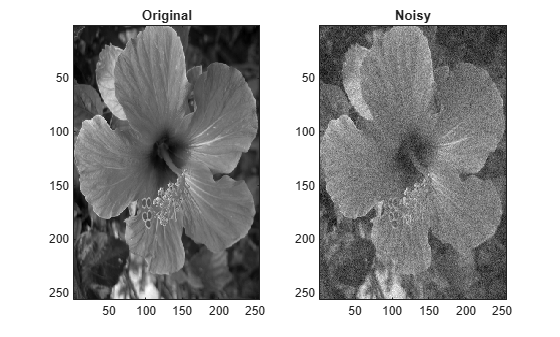Denoise the noisy image using the default `wdenoise2` settings. Compare with the original image.

```imden = wdenoise2(flower.Noisy); subplot(1,2,1) imagesc(imden) title('Denoised') subplot(1,2,2) imagesc(flower.Noisy) title('Noisy') colormap gray```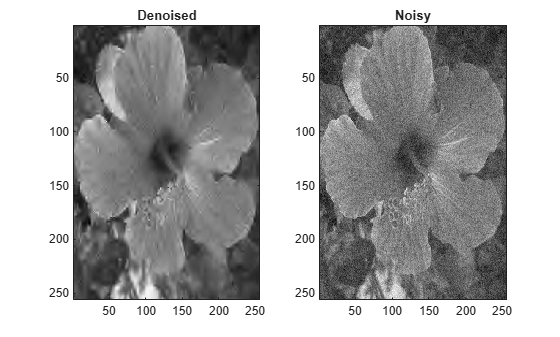Note the improvement in SNR before and after denoising.

```beforeSNR = ... 20*log10(norm(flower.Orig(:))/norm(flower.Orig(:)-flower.Noisy(:)))```
```beforeSNR = 14.1300 ```
```afterSNR = ... 20*log10(norm(flower.Orig(:))/norm(flower.Orig(:)-imden(:)))```
```afterSNR = 20.1388 ```

This example shows how to denoise a color image using cycle spinning.

Load the structure `colorflower`. The structure contains the RGB image of a flower, and a noisy version of that image. Display the original and noisy images.

```load colorflower subplot(1,2,1) imagesc(colorflower.Orig) title('Original') subplot(1,2,2) imagesc(colorflower.Noisy) title('Noisy')```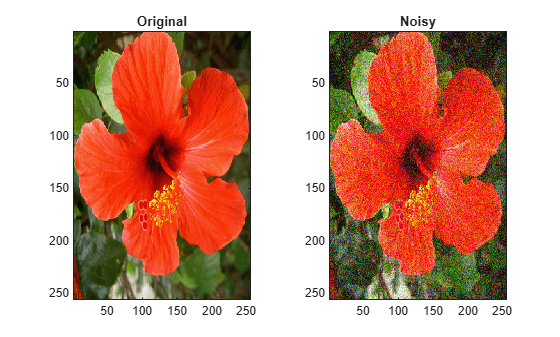Denoise the image down to level 2 using the default Bayesian method and cycle spinning with${\left(1+1\right)}^{2}$ shifts. Display the noisy and denoised images.

```imden = wdenoise2(colorflower.Noisy,2,'CycleSpinning',1); figure subplot(1,2,1) imagesc(imden) title('Denoised') subplot(1,2,2) imagesc(colorflower.Noisy) title('Noisy')```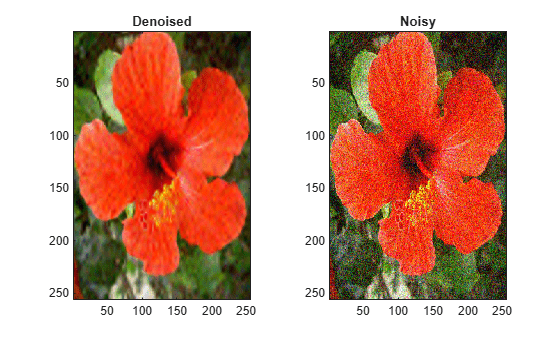Compute the SNR before and after denoising.

```beforeSNR = ... 20*log10(norm(colorflower.Orig(:))/norm(colorflower.Orig(:)-colorflower.Noisy(:)))```
```beforeSNR = 11.2217 ```
```afterSNR = ... 20*log10(norm(colorflower.Orig(:))/norm(colorflower.Orig(:)-imden(:)))```
```afterSNR = 19.8813 ```

This example shows how to denoise an image using a specific subband to estimate the variance of the noise.

Load the structure `flower`. The structure contains the grayscale image of a flower, and a noisy version of that image. Display the original and noisy images.

```load flower subplot(1,2,1) imagesc(flower.Orig) title('Original') subplot(1,2,2) imagesc(flower.Noisy) title('Noisy') colormap gray```Denoise the image down to level 2 using the False Discovery Rate method with a Q-value of 0.01. Denoise only based on the diagonal wavelet coefficients. Display the denoised and noisy images.

```imden = wdenoise2(flower.Noisy,2,... 'DenoisingMethod',{'FDR',0.01},... 'NoiseDirection',"d"); figure subplot(1,2,1) imagesc(imden) title('Denoised') subplot(1,2,2) imagesc(flower.Noisy) title('Noisy') colormap gray```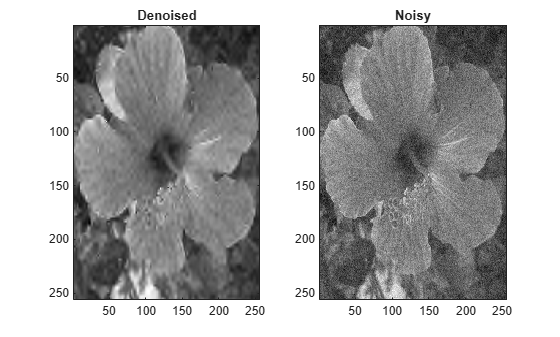Compute the SNR before and after denoising.

```beforeSNR = ... 20*log10(norm(flower.Orig(:))/norm(flower.Orig(:)-flower.Noisy(:)))```
```beforeSNR = 14.1300 ```
```afterSNR = ... 20*log10(norm(flower.Orig(:))/norm(flower.Orig(:)-imden(:)))```
```afterSNR = 14.1300 ```

## Input Arguments

collapse all

Input image to denoise, specified as a real-valued 2-D matrix or real-valued 3-D array. If `IM` is 3-D, `IM` is assumed to be a color image in the RGB color space and the third dimension of `IM` must be 3. For RGB images, `wdenoise2` by default projects the image onto its PCA color space before denoising. To denoise an RGB image in the original color space, use the `ColorSpace` name-value pair.

Wavelet decomposition level used for denoising, specified as a positive integer. `LEVEL` is a positive integer less than or equal to `floor(log2(min([M N])))` where `M` and `N` are the row and column sizes of the image. If unspecified, `LEVEL` defaults to the minimum ```floor(log2(min([M N])))``` and `wmaxlev([M N],wname)` where wname is the wavelet used (`'bior4.4'` by default).

### Name-Value Pair Arguments

Specify optional comma-separated pairs of `Name,Value` arguments. `Name` is the argument name and `Value` is the corresponding value. `Name` must appear inside quotes. You can specify several name and value pair arguments in any order as `Name1,Value1,...,NameN,ValueN`.

Example: `'NoiseEstimate','LevelDependent','Wavelet','sym6'`

Name of wavelet, specified as a character vector or string scalar, to use for denoising. The wavelet must be orthogonal or biorthogonal. Orthogonal and biorthogonal wavelets are designated as type 1 and type 2 wavelets respectively in the wavelet manager, `wavemngr`.

• Valid built-in orthogonal wavelet families begin with `'haar'`, `'dbN'`, `'fkN'`, `'coifN'`, or `'symN'` where `N` is the number of vanishing moments for all families except `'fk'`. For `'fk'`, `N` is the number of filter coefficients.

• Valid biorthogonal wavelet families begin with `'biorNr.Nd'` or `'rbioNd.Nr'`, where `Nr` and `Nd` are the number of vanishing moments in the reconstruction (synthesis) and decomposition (analysis) wavelet.

Determine valid values for the vanishing moments by using `waveinfo` with the wavelet family short name. For example, enter `waveinfo('db')` or `waveinfo('bior')`. Use `wavemngr('type',WNAME)` to determine if a wavelet is orthogonal (returns 1) or biorthogonal (returns 2).

Denoising method to use to determine the denoising thresholds for the image `IM`.

• `Bayes` - Empirical Bayes

This method uses a threshold rule based on assuming measurements have independent prior distributions given by a mixture model. Because measurements are used to estimate the weight in the mixture model, the method tends to work better with more samples. By default, the posterior median rule is used to measure risk .

• `FDR` - False Discovery Rate

This method uses a threshold rule based on controlling the expected ratio of false positive detections to all positive detections. The `FDR` method works best with sparse data. Choosing a ratio, or Q-value, less than `1/2` yields an asymptotically minimax estimator .

Note

For `'FDR'`, there is an optional argument for the Q-value, which is the proportion of false positives. Q is a real-valued scalar between `0` and `1/2`, ```0 < Q <= 1/2```. To specify `'FDR'` with a Q-value, use a cell array where the second element is the Q-value. For example, `'DenoisingMethod',{'FDR',0.01}`. If unspecified, Q defaults to `0.05`.

• `Minimax` - Minimax Estimation

This method uses a fixed threshold chosen to yield minimax performance for mean squared error against an ideal procedure. The minimax principle is used in statistics to design estimators. See `thselect` for more information.

• `SURE` - Stein's Unbiased Risk Estimate

This method uses a threshold selection rule based on Stein’s Unbiased Estimate of Risk (quadratic loss function). One gets an estimate of the risk for a particular threshold value (t). Minimizing the risks in (t) gives a selection of the threshold value.

• `UniversalThreshold` - Universal Threshold $\sqrt{2\mathrm{ln}\left(\text{length}\left(x\right)\right)}.$

This method uses a fixed-form threshold yielding minimax performance multiplied by a small factor proportional to `log(length(X))`.

Threshold rule to use to shrink the wavelet coefficients. `'ThresholdRule'` is valid for all denoising methods, but the valid options and defaults depend on the denoising method. Rules possible for different denoising methods are specified as follows:

• `'SURE'`, `'Minimax'`, `'UniversalThreshold'`: Valid options are `'Soft'` or `'Hard'`. The default is `'Soft'`.

• `'Bayes'`: Valid options are `'Median'`, `'Mean'`, `'Soft'`, or `'Hard'`. The default is `'Median'`.

• `'FDR'`: The only supported option is `'Hard'`. You do not need to define `'ThresholdRule'` for `'FDR'`

Method of estimating variance of noise in the image. Valid options are `'LevelIndependent'` and `'LevelDependent'`.

• `'LevelIndependent'` estimates the variance of the noise based on the finest-scale (highest-resolution) wavelet coefficients.

• `'LevelDependent'` estimates the variance of the noise based on the wavelet coefficients at each resolution level.

There are three wavelet subbands: horizontal, vertical, and diagonal. The value of `'``NoiseDirection``'` specifies which subbands to use in estimating the variance.

Wavelet subbands to use to estimate the variance of the noise, specified as a string vector or scalar string. Valid entries are `"h"`, `"v"`, or "d", for the horizontal, vertical, and diagonal subbands, respectively.

Example: `'NoiseDirection',["h" "v"]` specifies the horizontal and vertical subbands.

Number of circular shifts in both the row and column directions to use for denoising `IM` with cycle spinning. In cycle spinning, circular shifts of the image along the row and column dimensions are denoised, shifted back, and averaged together to provide the final result.

Generally, SNR improvements are observed with cycle spinning up to 3-4 shifts and asymptote after that. Because of the asymptotic effect on SNR and the fact that `(CycleSpinning+1)2` images are being denoised, it is recommended to start with `CycleSpinning` equal to 0. Then gradually increase the number of shifts to determine if there is any improvement in SNR to justify the computational expense.

For example, specifying `'CycleSpinning',1` results in four copies of `IM` being denoised:

• The original image (unshifted)

• `IM` circularly shifted a single-element along the row dimension

• `IM` circularly shifted a single-element along the column dimension

• `IM` circularly shifted a single-element along both the row and column dimensions

The four denoised copies of `IM` are denoised, reconstructed, shifted back to their original positions, and averaged together. The value of `CycleSpinning` represents the maximum shift along both the row and column dimensions. For RGB images, there are no shifts applied along the color space dimension.

Color space used for denoising an RGB image. Valid options are `'PCA'` and `'Original'`.

• `'PCA'`: The RGB image is first projected onto its PCA color space, denoised in the PCA color space, and returned to the original color space after denoising.

• `'Original'`: Denoising is done in the same color space as the input image.

`ColorSpace` is valid only for RGB images.

## Output Arguments

collapse all

Denoised image, returned as a matrix. The dimensions of `IM` and `IMDEN` are equal.

Scaling and denoised wavelet coefficients of the denoised image, returned as a real-valued matrix. `DENOISEDCFS` is a `(numshifts+1)2`-by-`N` matrix where `N` is the number of wavelet coefficients in the decomposition of `IM` and `numshifts` is the value of `'``CycleSpinning``'`. Each row of `DENOISEDCFS` contains the denoised wavelet coefficients for one of `(numshifts+1)2` shifted versions of `IM`. For RGB images, `DENOISEDCFS` are the denoised coefficients in the specified color space.

The ith row of `DENOISEDCFS` contains the denoised wavelet coefficients of the image circularly shifted by the amount returned in the ith column of `SHIFTS`. For example, if the second column of `SHIFTS` is `[1 ; 1]`, the second row of `DENOISEDCFS` contains the denoised coefficients of the image circularly shifted by a single element in the row direction and a single element in the column direction.

Scaling and wavelet coefficients of the input image, returned as a real-valued 2-D matrix. `ORIGCFS` is a `(numshifts+1)2`-by-`N` matrix where `N` is the number of wavelet coefficients in the decomposition of `IM` and `numshifts` is the value of the `'``CycleSpinning``'`. Each row of `ORIGCFS` contains the wavelet coefficients for one of `(numshifts+1)2` shifted versions of `IM`. For RGB images, `ORIGCFS` are the original coefficients in the specified color space.

The ith row of `ORIGCFS` contains the wavelet coefficients of the image circularly shifted by the amount returned in the ith column of `SHIFTS`. For example, if the second column of `SHIFTS` is `[1 ; 1]`, the second row of `ORIGCFS` contains the coefficients of the image circularly shifted by a single element in the row direction and a single element in the column direction.

Bookkeeping matrix. The matrix `S` contains the dimensions of the approximation coefficients at the coarsest scale, the sizes of the wavelet coefficients at all scales, and the size of the original input image. `S` is a matrix with the same structure as the `S` output of `wavedec2`.

Image shifts used in cycle spinning, returned as an integer-valued matrix. `SHIFTS` is 2-by-`(numshifts+1)2` matrix where each column of `SHIFTS` contains the shifts along the row and column dimension used in cycle spinning.

 Abramovich, F., Y. Benjamini, D. L. Donoho, and I. M. Johnstone. “Adapting to Unknown Sparsity by Controlling the False Discovery Rate.” Annals of Statistics, Vol. 34, Number 2, pp. 584–653, 2006.

 Antoniadis, A., and G. Oppenheim, eds. Wavelets and Statistics. Lecture Notes in Statistics. New York: Springer Verlag, 1995.

 Donoho, D. L. “Progress in Wavelet Analysis and WVD: A Ten Minute Tour.” Progress in Wavelet Analysis and Applications (Y. Meyer, and S. Roques, eds.). Gif-sur-Yvette: Editions Frontières, 1993.

 Donoho, D. L., I. M. Johnstone. “Ideal Spatial Adaptation by Wavelet Shrinkage.” Biometrika, Vol. 81, pp. 425–455, 1994.

 Donoho, D. L. “De-noising by Soft-Thresholding.” IEEE Transactions on Information Theory, Vol. 42, Number 3, pp. 613–627, 1995.

 Donoho, D. L., I. M. Johnstone, G. Kerkyacharian, and D. Picard. “Wavelet Shrinkage: Asymptopia?” Journal of the Royal Statistical Society, series B, Vol. 57, No. 2, pp. 301–369, 1995.

 Johnstone, I. M., and B. W. Silverman. “Needles and Straw in Haystacks: Empirical Bayes Estimates of Possibly Sparse Sequences.” Annals of Statistics, Vol. 32, Number 4, pp. 1594–1649, 2004.

## SupportGet trial now